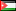##### “A comparison Study Among Selected Equations For Predicting Resting Metabolic Rate of Volleyball Players”
Publication Type
Original research
Authors
Fulltext

“A comparison Study Among Selected Equations For Predicting Resting Metabolic Rate of Volleyball Players”

The Seventh International Conference, 29-30/4/2015, Faculty of Physical Education, Yarmok University.

The purpose of this study was to examine the differences of four published equations for predicting (RMR) kcal/ day in male volleyball players, and determine the efficacy of Body Surface Area (BSA) in the prediction of (RMR) . The sample consisted of (101) players from different grades in Palestine. (DeLorenzo et al., 1999), (Mifflin et al., 1990), (WHO, 1985) and (Harris & Benedict, 1919) equations were used in measuring (RMR). The results of (MANOVA) using Hotelling’s Trace Test, and Sidak post-hoc test indicated a significant differences at (α = 0.05) among all equations. The highest mean was for using (DeLorenzo et al. , 1999) equation (1930.38)kcal/day, while the lowest mean was for (Mifflin et al., 1990) equation (1777.95) kcal/day. Also, the results revealed high correlation between (BSA) and (RMR),   The regression  equation was:

(RMR) kcal/day = (-59.666) +((1024.402)*(BSA Meter Square ))

(R2= 0.962; SE= 20 kcal/day, Beta = 0.99)

Based on the study finding the researcher recommended to conduct other research studies for different games and sport events. In addition, studying the correlation between anthropometric measures and (RMR) for athletes.

Journal
Title
عبد الناصر القدومي
Publisher
المؤتمر الدولي السابع-كلية التربية الرياضية-جامعة اليرموك.2015
Publisher Country
JordanPublication Type
Both (Printed and Online)
Volume
1
Year
2015
Pages
1